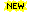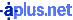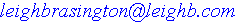The Factors of the Gradual Training As Found in Various Suttas

X = Factor occurs in the sutta(s)     I = Insight practice, but not usual stock passage     L = Liberation indicated, but not usual stock passage
GF SmlM MedM LrgM V GS ME DtW M&CC CwL 5H 4EM BV J1 J2 J3 J4 J5 J6 J7 J8 N I MMB SNP DEar KMO RPL SRAK EndA   DN 2, 3, 4, 5, 8 30     Factors        32  Suttas X X X X X X X X X X X X X X X X X X X X X * X X X X X X X X X X X X X X X X X X X X X X X X X X X X X X X X X X X X X X X X X X X X X X X X X X X X X X X X X X X X X X X X X X X X X X X X X X X X X X X X X X X † X X X X X X X X X X I L X X X X X X X X X X X X X X X X X X X X X X X X X X X X X X X X X X X X X X X X X X X X X X X ‡ X X X X X X X X X X X X X X X X X X X X X X X X X X X X X X X X X X X X X X X X X X X X X X X X X X X X X X X X X X X X X X X X X X X X X X X X X X X X (Place your cursor on theletters in a column headingto see what they stand for,or scroll down) 24 23 8 8 27 28 4 4 27 23 29 1 1 30 31 31 31 2 2 2 1 2 12 8 7 7 7 19 19 29 4 Fac in 30 Su (94%) X X X X 6 Fac in 29 Su (91%) X X X X X X 9 Fac in 27 Su (84%) X X X X X X X X X 12 Fac in 23 Su (72%) X X X X X X X X X X X X ‡ 14 Fac in 19 Su (59%) X X X X X X X X X X X X X X † 15 Fac in 12 Su (38%) X X X X X X X X X X X X X X X 17 Fac in 8 Su (25%) X X X X X X X X X X X X X X X X X 21 Fac in 7 Su (22%) X X X X X X X X X X X X X X X X X X X X X * 9 Fac in < 5 Su (<16%) X X X X X X X X X * = Same 21 factors as in DN 2, etc.       † = Same 14 factors as in MN 27, etc.       ‡ = Same 12 factors as in MN 112 The height of the barfor each factor isproportional to thenumber of the 32 suttasthat factor appears in. Grouping Color Key: Going Forth Precepts   More Sīla Hindrances   Jhanas Insight Practice Psychic Powers Awakening Other ████████████ ▄███████████ ████ ████ ▄█████████████ ██████████████ ██ ██ ▄█████████████ ▄███████████ ▄██████████████ ▄ ▄ ███████████████ ▄███████████████ ▄███████████████ ▄███████████████ █ █ █ ▄ █ ██████ ████ ▄███ ▄███ ▄███ ▄█████████ ▄█████████ ▄██████████████ 24 23 8 8 27 28 4 4 27 23 29 1 1 30 31 31 31 2 2 2 1 2 12 8 7 7 7 19 19 29 24 66 86 29 1 1 130 2 12 66 29 152 163 107

Abrv#Factor Description
GF24a tathāgata arises in this world, one hears the dhamma, Gains Faith, Goes Forth into homelessness
SmlM23Small section on Morality
MedM8Medium section on Morality
LrgM8Large section on Morality
V27Virtue summarized
GS28Guarding the Senses
ME4Moderation in Eating
DtW4Devotion to Wakefulness
M&CC27Mindfulness and Clear Comprehension (sati & sampajañña)
CwL23Contentment with Little - food, clothing, shelter, medicine when ill
5H29abandoning the 5 Hindrances - sensual desire, hatred & ill-will, restless & remorse, sloth & torpor, doubt
4EM14 Establishments of Mindfulness (4 satipaṭṭhāna)
BV1practicing the 4 Brahama Viharas (mettā, karunā, muditā, upekkhā)
J1301st Jhana
J2312nd Jhana
J3313rd Jhana
J4314th Jhana
J525th Jhana - infinite space
J626th Jhana - infinite consciousness
J727th Jhana - nothingness
J818th Jhana - neither perception nor non-perception
N2Nirodha - the cessation of feeling and perception
I12Insight practice
SNP7SuperNormal Powers
DEar7Divine Ear
KMO7Knowing the Minds of Others
RPL19Remembering Past Lives
SRAK19Seeing beings passing away and Rearising According to their Karma
EndA29the Ending of the Āsavas - Liberation

View this Chart as a JPG
Sekha Paṭipadā Chart another similar chart, tho apparently using a different version of the PaliThe Similes from the Gradual Training
Back to Suttas
Back to Essays
Permalink http://leighb.com/gtchart.htm [] Hosted byLeigh Brasington // Revised 09 Nov 22